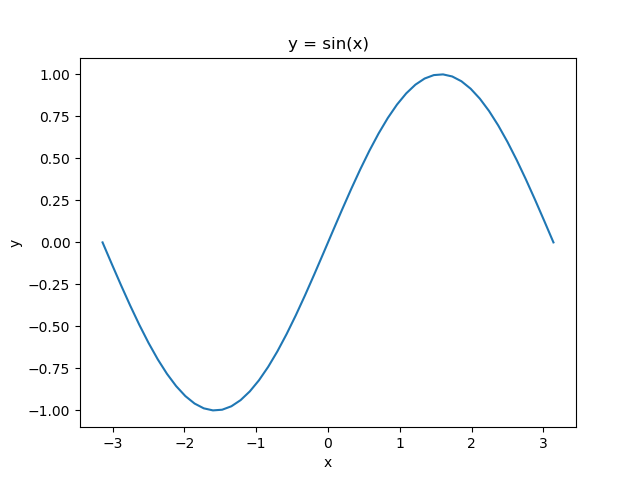# [python] A note that started to understand the behavior of matplotlib.pyplot

Pyplot, a module for drawing graphs, is sometimes taken care of, but I was not accustomed to using it. I've finally been able to eliminate that haze recently, so I'll leave it as a note of my awareness.

• The content is not "why it is designed like that" but "what kind of design it is".

## Where I didn't understand personally

First is the basic graph output.

``````import matplotlib.pyplot as plt
import numpy as np

x = np.linspace(-np.pi, np.pi)
y = np.sin(x)

fig = plt.figure()
plt.plot(x, y)
plt.title("y = sin(x)")
plt.xlabel("x")
plt.ylabel("y")
fig.savefig("output.png ")
``````With this, the image is output as ʻoutput.png`, but personally, the behavior at this time did not fall into my mind. For example

--There is no evidence of creating a `plt` instance --I used the `plt` method to change the title of the graph, but I called the` fig` method to save it as an image. --The target when accessing with `plt` just by generating` fig1`, `fig2`, ... like` fig` changes in order as `fig1`,` fig2`, ...

(By the way, I didn't think that `title ()` and `xlabel ()` are class methods). Personally, I'm used to the flow of instance creation → method call, so I think I understood without hesitation if the method was as below.

``````#This does not work properly.
import matplotlib
import numpy as np

x = np.linspace(-np.pi, np.pi)
y = np.sin(x)

plt = matplotlib.pyplot() #I want you to create an instance here
plt.plot(x, y)
plt.title("y = sin(x)")
plt.xlabel("x")
plt.ylabel("y")
plt.figure.savefig("output.png ") #I want this to be a method of the plt instance
``````

Why is it successful in the first way? And why doesn't it work the way I envisioned?

## What's really going on

Find out who each of the elements in the first working script is (omip the numpy part).

• `matplotlib` --Library for drawing graphs
• `pyplot` --Modules in the `matplotlib` library --ʻImport Import and use with matplotlib.pyplot` etc.
• `plt` --Alias for `matplotlib.pyplot` (hence type is module) --Since it is an alias, you can change it to any name you like.
• `fig` --An instance of the `matplotlib.figure.Figure` class --Generated by `fig = pyplot.figure ()`

The important thing is that ** `plt` is a module **, not a class. If `plt` was a class, I would like it to be instantiated somewhere, but because it was a module, it was already created when I imported it on the second line, so suddenly`fig = plt.figure ()`and`It worked fine with plt.plot (x, y)`. Also, this is speculation, but if you think that the access destination is updated in `plt` when the` fig` instance is created, other questions may be convincing.

## bonus

Now, in python, modules are created as singletons, so they refer to objects with the same id no matter where you call them. Therefore, if you call `pyplot` elsewhere, you will still be able to access the graph you have already created.

For example, suppose the following two files are in the same directory:

#### `graph.py`

``````
import matplotlib.pyplot as plt
import numpy as np

def edit_graph():
x = np.linspace(-np.pi, np.pi)
y = np.sin(x)

plt.plot(x, y)
plt.title("y = sin(x)")
plt.xlabel("x")
plt.ylabel("y")
``````

#### `main.py`

``````
import matplotlib.pyplot as plt_main
import graph

graph.edit_graph()
plt_main.show()
``````

If you execute `python main.py` in this state, the same sine wave graph as before will be output. In other words, it is possible to output a graph generated / edited in one script file in another script file without creating / passing an instance (although it is not recommended by design). You can also call the method `savefig ()` in the `matplotlib.figure.Figure` class after executing`fig = pyplot.figure ()`.

## Summary

The main reason for this question was that I misunderstood `plt` as some kind of class or instance. I don't know why I thought so now ... It's not a big deal if you realize that `plt` is a module, but I think it will be better understood if you think about it again. ..

Finally, a summary of what I noticed this time.

--`pyplot` is a module and is usually used with a name like` plt`. --The `pyplot` module itself behaves like a class or its instance --Every time you execute `fig = pyplot.figure ()`, a `fig` instance of the` matplotlib.figure.Figure` class is created, and each time you execute it, the access target by the `pyplot` module changes.